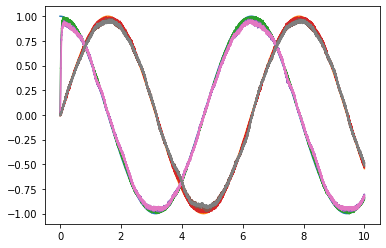# Ensemble array¶

An ensemble array is a group of ensembles that each represent a part of the overall signal.

Ensemble arrays are similar to normal ensembles, but expose a slightly different interface. Additionally, in an ensemble array, the components of the overall signal are not related. As a result, network arrays cannot be used to compute nonlinear functions that mix the dimensions they represent.

:

%matplotlib inline
import matplotlib.pyplot as plt
import numpy as np

import nengo

:

model = nengo.Network(label="Ensemble Array")
with model:
# Make an input node
sin = nengo.Node(output=lambda t: [np.cos(t), np.sin(t)])

# Make ensembles to connect
A = nengo.networks.EnsembleArray(100, n_ensembles=2)
B = nengo.Ensemble(100, dimensions=2)
C = nengo.networks.EnsembleArray(100, n_ensembles=2)

# Connect the model elements, just feedforward
nengo.Connection(sin, A.input)
nengo.Connection(A.output, B)
nengo.Connection(B, C.input)

# Setup the probes for plotting
sin_probe = nengo.Probe(sin)
A_probe = nengo.Probe(A.output, synapse=0.02)
B_probe = nengo.Probe(B, synapse=0.02)
C_probe = nengo.Probe(C.output, synapse=0.02)

:

with nengo.Simulator(model) as sim:
sim.run(10)

:

# Plot the results
plt.figure()
plt.plot(sim.trange(), sim.data[sin_probe])
plt.plot(sim.trange(), sim.data[A_probe])
plt.plot(sim.trange(), sim.data[B_probe])
plt.plot(sim.trange(), sim.data[C_probe])

:

[<matplotlib.lines.Line2D at 0x7f81c3b57358>,
<matplotlib.lines.Line2D at 0x7f81c3b57748>]These plots demonstrate that the ensemble array works very similarly to a standard N-dimensional population. However, this is not true when it comes to computing functions. Ensemble arrays cannot be used to compute nonlinear functions that mix the dimensions they represent.